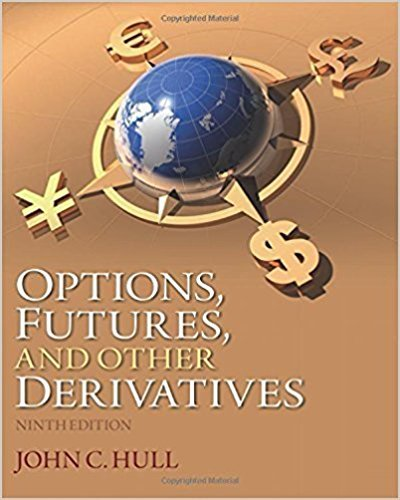×
×

# Solutions for Chapter 31: Interest Rate Derivatives: Models of the Short Rate## Full solutions for Options, Futures, and Other Derivatives | 9th Edition

ISBN: 9780133456318Solutions for Chapter 31: Interest Rate Derivatives: Models of the Short Rate

Solutions for Chapter 31
4 5 0 349 Reviews
26
5
##### ISBN: 9780133456318

Since 28 problems in chapter 31: Interest Rate Derivatives: Models of the Short Rate have been answered, more than 15286 students have viewed full step-by-step solutions from this chapter. Chapter 31: Interest Rate Derivatives: Models of the Short Rate includes 28 full step-by-step solutions. Options, Futures, and Other Derivatives was written by and is associated to the ISBN: 9780133456318. This textbook survival guide was created for the textbook: Options, Futures, and Other Derivatives, edition: 9. This expansive textbook survival guide covers the following chapters and their solutions.

Key Business Terms and definitions covered in this textbook
• accounting profit

total revenue minus total explicit cost

• agent

a person who is performing an act for another person, called the principal

fluctuations in economic activity, such as employment and production

• common resources

goods that are rival in consumption but not excludable

• consumer surplus

the amount a buyer is willing to pay for a good minus the amount the buyer actually pays for it

• diversification

the reduction of risk achieved by replacing a single risk with a large number of smaller, unrelated risks

• efficiency

the property of society getting the most it can from its scarce resources

• equality

the property of distributing economic prosperity uniformly among the members of society

• equilibrium

a situation in which the market price has reached the level at which quantity supplied equals quantity demanded

• financial system

the group of institutions in the economy that help to match one person’s saving with another person’s investment

• marginal cost

the increase in total cost that arises from an extra unit of production

the costs of changing prices

• perfect substitutes

two goods with straight-line indifference curves

• price elasticity of supply

a measure of how much the quantity supplied of a good responds to a change in the price of that good, computed as the percentage change in quantity supplied divided by the percentage change in price

• profit

total revenue minus total cost

• real exchange rate

the rate at which a person can trade the goods and services of one country for the goods and services of another

• regressive tax

a tax for which highincome taxpayers pay a smaller fraction of their income than do low-income taxpayers

• social insurance

government policy aimed at protecting people against the risk of adverse events

• welfare

government programs that supplement the incomes of the needy welfare economics the study of how the allocation of resources affects economic well-being

• world price

the price of a good that prevails in the world market for that good

×